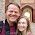## Sunday, September 9, 2012

### Matrix Operations in Mata

* This post demonstrates a few methods for how to input matrices into Mata and how to do some basic matrix operations.  Mata is a matrix programming language so basic matrix operations are extremely easy.

mata
// Let's first build some matrices in Mata
// They can be built directly
A = ( 2 , -1, 5 \ 3, 0 , -1 \ 3, 3 , -1)
A

B1 = (0 , 3 ,-1)
B2 = (3 , 0 , 0)
B3 = (0 , 2 , 0)

// Or through a combination of vectors
B = (B1 \ B2 \ B3)

B

// We can also start with an empty matrix and fill in values

C = J(3,2,4)
// The J command creates a matrix with 3 rows, 2 colums, and with default values of 4

C
// We can replace individual elements once the matrix is created
C[1,2] = 3
C[3,1] = 7

// Or entire submatrices
C[2,] = (2,6)
C

// Now let's see how various matrix operations perform in Mata:

// a. AB
A*B

// b. BA
B*A

// c. A+B
A+B

// d. A'B'
A'*B'

// e. B'A'
B'*A'

// f. A'B
A'*B

// g. (AB)'
(A*B)'

// h. ABC
A*B*C

// i. C'AC
C'*A*C

// j. CAC'
C*A*C'
// This does not exist because C*A cannot be multiplied as C is 3x2 and A is 3x3

// k. trace(C'AC)
trace(C'*A*C)

// l. trace(CAC')
trace(C*A*C')
// Likewise the trace does not exist

end

* Matrices can also be input into Mata from data sets.

clear
set obs 5
gen y = 3
replace y = 6  if _n == 2
replace y = 10 if _n == 3
replace y = 8  if _n == 4
replace y = 2  if _n == 5

mata
// The command st_data retrieves data from stata variables to be used in Mata
y = st_data(. , "y")
y

// Once input, matrices can easily be manipulated
y*y'

// The square of the norm of y
norm(y)^2

// This happens to be identical to:
sum(y:^2)

// The norm is the equclidean norm which is the square square root of the sum of all of the squares of a vector.
// Thus the square of it is just the sum of the squares.

// As should be clear, manipulating matrices in Mata is extremely easy.
// Thus Stata is able to pack a powerful Matrix programming language inside an effective high level user language.

// The largest frustration that I have had with Mata is the relative quality of the documentation.
// I find the documentation of Mata much harder to use than that of Stata (at least in version 11, perhaps version 12 has better documentation).
end

1.Do you know how to turn a Mata matrix into a Stata matrix? I'm basically looking for the opposite of "st_matrix()".

1.Interestingly, st_matrix works either direction. It just depends which argument you feed it.

st_matrix("B_shell", B)

from Mata will send the B matrix into the Stata shell and call it B_shell. You can see it:

matrix list B_shell